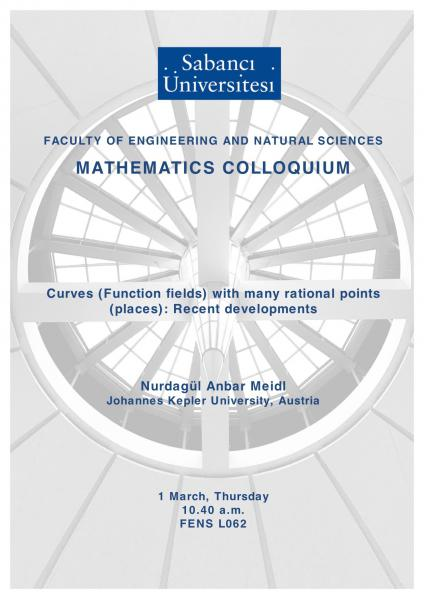## Mathematics Colloquium: Curves (Function fields) with many rational po..

### Mathematics Colloquium: Curves (Function fields) with many rational po..You are cordially invited to attend the colloquium given by Nurdagül Anbar Meidl (Johannes Kepler University, Austria) on Thursday, 1 March 2018, in FENS-L062 at 10.40 am.

Speaker: Nurdagül Anbar Meidl

Title: Curves (Function fields) with many rational points (places): Recent developments

Date/Time:  Thursday, 1 March @ 10:40 am

Place: FENS - L062

Abstract: Let Fbe the finite field with q elements. X denotes an absolutely irreducible, projective curve defined over Fq. Having many applications in other branches of mathematics, special interest arises on the question how many rational points (i.e., the points with coordinates in Fq) X can have. Hasse and Weil showed that the number N(X) of rational points of the curve X is bounded by q and an invariant g(X) attached to the curve (which is called genus); namely N(X)≤1+q+2g(X)√q .This bound is called the Hasse–Weil Bound. Then Ihara observed that this bound is not optimal when the genus is large compared to the cardinality of the finite field q. This observation led to the investigation of the number of rational points of curves of large genus, and resulted in Ihara’s constant A(q) defined by A(q):=limsup N(X)/g(X) where limsup is taken over all curves defined over Fwith genus tending to infinity. In this talk, I will briefly describe curves over finite fields, their number of rational points, Ihara’s constant and discuss recent developments.

Contact: Yasemin Şengül Tezel